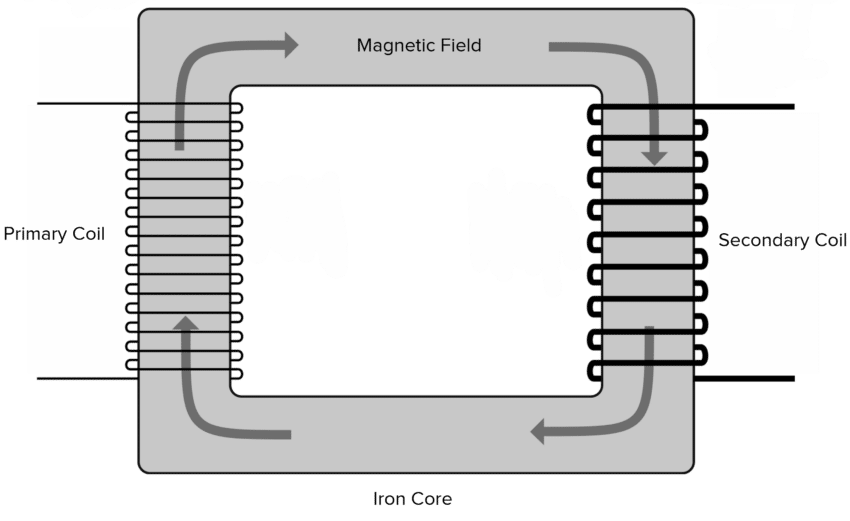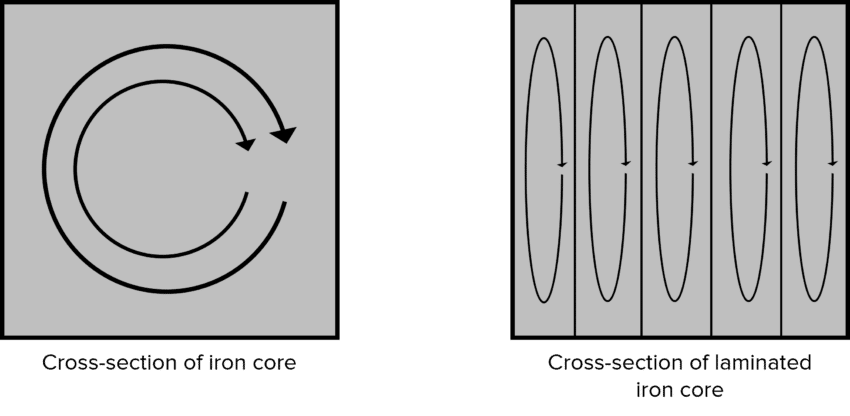# Transformers

A LevelAQA

## Transformers

Transformers are essential to transform the current and voltage of the power supply to ones that are suitable for the device.

## Transformers

A transformer consists of a soft iron core and two coils of wire. The coils are known as the primary coil and the secondary coil. The current enters the primary coil and causes a magnetic field to flow around the soft iron core. As the changing magnetic field reaches the secondary coil, an EMF is induced in the secondary coil.Although the transformer changes the voltage, the frequency of the alternating current is not affected by the transformer.

The transformer equation states:

$\dfrac{N_s}{N_p} = \dfrac{V_s}{V_p}$

• $N_s$ is the number of turns on the secondary coil.
• $N_p$ is the number of turns on the primary coil.
• $V_s$ is the voltage at the secondary coil in volts $\left(\text{V}\right)$.
• $V_p$ is the voltage at the primary coil in volts $\left(\text{V}\right)$.

Example: A transformer changes a $230 \: \text{V}$ power supply to $100 \: \text{V}$ output. If the primary coil has $120$ turns, how many turns are on the secondary coil?

[2 marks]

$\dfrac{N_s}{N_p} = \dfrac{V_s}{V_p}$

$\dfrac{N_s}{120} = \dfrac{100}{230}$

$N_s = \dfrac{100}{230} \times 120$

$N_s = 52 \: \text{turns}$

A transformer may either be a step-up or step-down transformer. A step-up transformer increases the voltage and a step-down transformer decreases the voltage supply.

The most common use of a step-up transformer is to increase the voltage (decrease the current) of a power supply for efficient transport in transmission cables. It always has more turns on the secondary coil than the first.

A step-down transformer reduces the voltage to a level that is safe enough to use in a device or certain area. They always have more turns on the primary coil than the secondary.

A LevelAQA

## Efficiency

As with almost all devices, transformers are not $100 \%$ efficient. Therefore the power output is always slightly less than the power input. Efficiency is a measure of how much of the energy input is transformed to useful energy output.

The transformers efficiency can be calculated by the equation:

## $\text{Efficiency} = \dfrac{I_s V_s}{I_p V_p}$

• $V_s$ is the voltage at the secondary coil in volts $\left(\text{V}\right)$.
• $V_p$ is the voltage at the primary coil in volts $\left(\text{V}\right)$.
• $I_s$ is the current of the secondary coil in amps $\left(\text{A}\right)$.
• $V_p$ is the current of the primary coil in amps $\left(\text{A}\right)$.
A LevelAQA

## Inefficiencies in Transformers

Transformers are not always $100 \%$ efficient. The majority of the energy loss in a transformer comes from the production of Eddy currents. When the magnetic field is induced in the iron core, a small EMF is also induced which acts opposite in direction to the change that caused it (Lenz’s law). The current flowing in the soft iron core causes energy to dissipate as heat.The Eddy currents can be reduced by laminating the iron core. This is where the iron core is sliced into smaller pieces with insulating materials wedged between the slices of iron. The higher the resistivity of metal also reduces eddy currents.

A LevelAQA

## Transformers Example Questions

To conduct magnetic fields between the primary coil and the secondary coil.

\begin{aligned} \dfrac{N_s}{N_p} &= \dfrac{V_s}{V_p}\\ \\ V_s &= \dfrac{N_s V_p}{N_p} \\ \\ \boldsymbol{V_s} &\boldsymbol{= \dfrac{500 \times 230}{120}} \\ \\ \boldsymbol{V_s} &\boldsymbol{= 958} \: \textbf{V} \end{aligned}

Eddy currents are hard to prevent. They form in the iron core and produce an electric current opposite the motion that caused them. They therefore increase power loss and decrease the efficiency of a transformer.

## Transformers Worksheet and Example Questions

### Transformers Questions

A LevelOfficial MME

## You May Also Like...### MME Learning Portal

Online exams, practice questions and revision videos for every GCSE level 9-1 topic! No fees, no trial period, just totally free access to the UK’s best GCSE maths revision platform.

£0.00